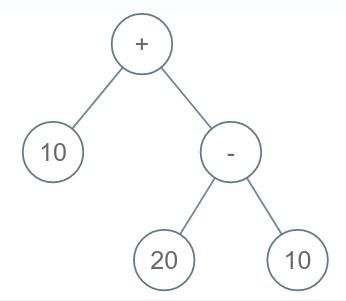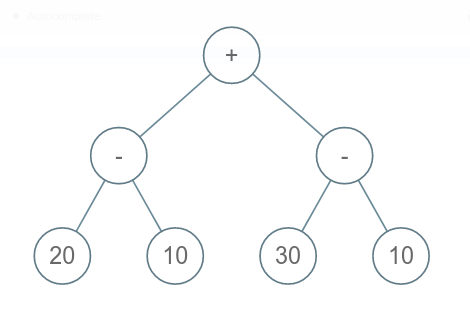# Evaluate Expression Tree

Posted: 11 Dec, 2020
Difficulty: Moderate

## PROBLEM STATEMENT

#### A binary tree is a tree where every node has at most two child nodes.

##### Note:
``````There can be 4 operators: +,-,*,/. For division, you need to return the floor(a/b).
``````
##### For example:
``````Consider the following expression tree:
````````````While evaluating the expression tree we replace the value at a node with any operation OP by evaluating LEFTCHILD (OP) RIGHTCHILD

Here, the expression will be evaluated as 20 - 10 = 10 (FOR ‘-’), then ‘-’ will be replaced by 10 (symbolic), then we evaluate for node’+’, 10 + 10 = 20.
``````
##### Input Format:
``````The first line of input contains an integer ‘T’ representing the number of test cases. Then the test cases follow.

The only line of each test case contains elements in the level order form. The line consists of values of nodes separated by a single space. In case a node is null, we take -1 in its place.

For example, the input for the tree depicted in the below image would be:
````````````+
- -
20 10 30 10
-1 -1 -1 -1 -1 -1 -1 -1
``````

#### Explanation:

``````Level 1:
The root node of the tree is ‘+’.

Level 2:
Left child of ‘+’ = ‘-’
Right child of ‘+’ = ‘-’

Level 3:
Left child of ‘-’ = 20
Right child of ‘-’ = 10
Left child of ‘-’ = 30
Right child of ‘-’ = 10

Level 4:
Left child of 20 = null (-1)
Right child of 20 = null(-1)
Left child of 10 = null (-1)
Right child of 10 = null (-1)
Left child of 30 = null (-1)
Right child of 30 = null (-1)
Left child of 10 = null (-1)
Right child of 10 = null (-1)

The first not-null node(of the previous level) is treated as the parent of the first two nodes of the current level. The second not-null node (of the previous level) is treated as the parent node for the next two nodes of the current level and so on.
The input ends when all nodes at the last level are null(-1).
``````
##### Note:
``````The above format was just to provide clarity on how the input is formed for a given tree.

The sequence will be put together in a single line separated by a single space. Hence, for the above-depicted tree, the input will be given as:

+ - - 20 10 30 10 -1 -1 -1 -1 -1 -1 -1 -1
``````
##### Output Format:
``````For each test case, the output will be the evaluated value for the expression tree.

The output for each test case will be in a separate line.
``````
##### Note:
``````You do not need to print anything; it has already been taken care of.
``````
##### Constraints:
``````1 <= T <= 100
1 <= N <= 2047
1 <= data <= 1000

Time Limit: 1 second
``````Approach 1

Consider function EVALUATEEXPRESSION that accepts as a parameter, a BinaryTreeNode of Strings, ROOT and do:

• If ROOT is NULL return 0.
• If both LEFT and RIGHT child of ROOT are NULL return value of ROOT as an integer.
• Define LEFTVAL. Call function EVALUATEEXPRESSION for a LEFT child of ROOT and assign its value to LEFTVAL.
• Define RIGHTVAL. Call function EVALUATEEXPRESSION for a RIGHT child of ROOT and assign its value to RIGHTVAL.
• If ROOT’s value is +, return LEFTVAL + RIGHTVAL
• If ROOT’s value is -, return LEFTVAL - RIGHTVAL
• If ROOT’s value is *, return LEFTVAL * RIGHTVAL
• If ROOT’s value is /, return LEFTVAL / RIGHTVAL